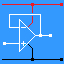RANDOM PAGE

SITE SEARCH

LOG
IN

HELP

# Voltage EMF PD

This is the AQA version closing after June 2019. Visit the the version for Eduqas instead.

a

## Electromotive Force (EMF) - Measured in Volts

• This is the mysterious force that tends to move charge in a circuit.
• The term EMF is usually applied to voltage sources like cells, batteries, dynamos, alternators, photo diodes and solar cells. These all produce electricity.
• The sum of the voltages in a series circuit is equal to the voltage across the whole circuit.
b

### The Voltmeter

• Voltmeters measure the potential difference (voltage) ACROSS a component.
• Connect the voltmeter in parallel with the device being measured.
• Ammeters measure the current THROUGH a component. Connect the ammeter in series with the device being measured.

Ideal voltmeters ...

• have infinite resistance
• no current flows through them
• do not disturb the circuit being measured

Real voltmeters come close to the ideal but are never perfect.

c

### Measuring Voltage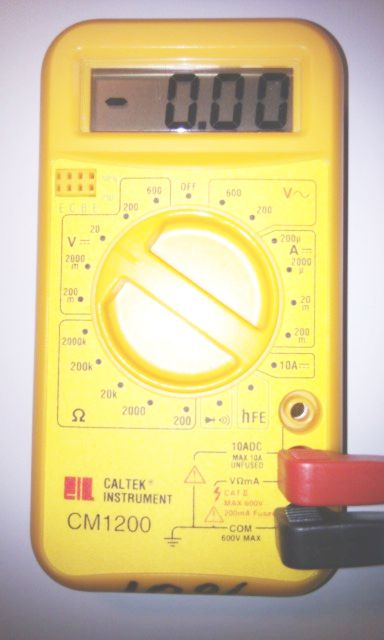Connect the voltmeter in parallel with the component who's potential difference you want to measure. The circuit below shows the battery voltage being measured and also the voltage across the bulb. (the same in this case)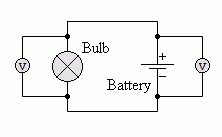d

### Polarity

This identifies the positive (+) and the negative (-) ends of components. Getting the polarity right can be very important. Electrolytic Capacitors and many semiconductors are destroyed if the polarity is wrong. The failure can be explosive.

e

### Potential and Potential Difference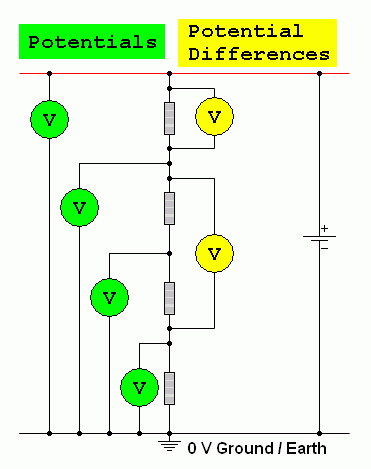f

### Potential

This is voltage measured relative to the the zero Volt ground potential.

g

### Potential Difference

This is the difference between two potentials. It is measured across components by connecting a Volt meter in parallel with the device to be measured.

h

### Voltage

This is just a slack usage of language. You should specify EMF, Potential or Potential Difference. As these concepts are all very similar, voltage is often used instead.

i

### The Volt

• One Joule of energy is exchanged when one Coulomb of charge is moved through a potential difference of one Volt.
• With some maths, you can prove that one volt is the potential difference across a conductor when a current of one ampere dissipates one watt of power.

For the Falstad Circuit Simulation, CTRL+Click Voltage Divider Simulation
In options, check European Resistors and uncheck Conventional Current.
Click the switch to turn the circuit on or off. Watch the voltages change.

Alternatively view Voltage_PD_EMF.txt.
Save or copy the text on the web page. Import the saved or copied text into the Falstad simulator.

Here is the new HTML5 Simulator Site.

j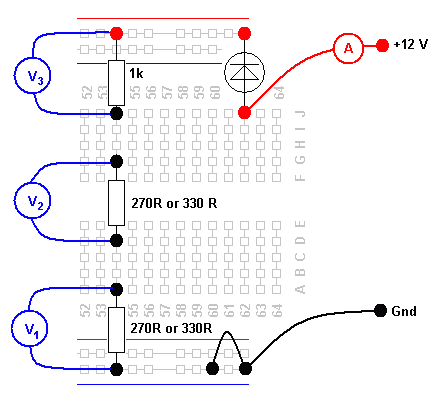Build this circuit and measure V1, V2 and V3. Also measure the current flowing.

• Calculate the resistance of each resistor using Ohm's law:        R = V / I